## Excel Dynamic Array Functions – What are they, how to use them, Examples and FAQs

Excel Dynamic Array Functions are a true game changer. These newly introduced DA functions can filter, sort, remove duplicates and do much more. The output of these functions can go to a range of cells. Hence the name – dynamic array functions.

## How Dynamic Array Functions differ from normal functions?

• Normal functions return value in ONE cell, where as DA functions return output to a range of cells

• New ways to work with data – FILTER, SORT, extract UNIQUE items and generate SEQUENCE of numbers or random values

• Can be use alone or with existing Excel functions

• Available in Excel 365 only

## What are the newly introduced DA functions?

As of April 2020, Microsoft introduced below 6 new functions under Dynamic Array category.

1. FILTER: to filter a range of cells (or table) based on input criteria
2. UNIQUE: to extract unique items from a a range of cells (or table)
3. SORT: to sort a list by a specified column.
4. SORTBY: to sort a list by another list
5. SEQUENCE: to generate a sequence of numbers in a range of rows and (or) columns.
6. RANDARRAY: to generate a range of random numbers

Here is a TLDR version of the Dynamic Array functions.Apart from these new functions, DA capability enables these extra features in Excel.

• You can use # operator to refer to a range of cells spilled by Dynamic Array functions. For example, if A1 has a DA function that returns 10×1 range (A1:A10), you can refer to this dynamic range by using the reference A1#
• Most array formulas will now simply spill into a range of cells. No need to press CTRL+SHIFT+Enter.
• Newly introduced formulas like XLOOKUP can also spill producing an entire row of matching result.
• Any formula or name that refers to more than one value will automatically spill. For example, if you type =data in a cell, it will return the entire table in a spilled range.

## Sample Data

I am using the below Employee data to demonstrate new Dynamic Array functions. This is in a table named “data”.## FILTER() Fact Sheet

What does FILTER() do?

FILTER() function filters a table or list of data based on conditions.

Show me a demo of FILTER?What is the syntax of FILTER function?

=FILTER(your data, conditions, if empty value)

Give me few examples of FILTER function?

Here are a few more examples of FILTER function:

• FILTER(data, data[manager]=”Ian”)
Shows all data where manager is Ian.
• FILTER(data, (data[age]>30)*(data[department]=”Website”))
All data where age > 30 AND department is Website.
• FILTER(data[name], (data[age]>30)+(data[department]=”Website”))
Show names where Age > 30 OR department is Website.
• FILTER(data, (data[Manager]<>”Ian”)*(data[gender]=”Female”))
Show female staff data where manager is NOT Ian

## UNIQUE() Fact Sheet

What does UNIQUE() do?

UNIQUE() function generates a list of unique items from input. You can use it to remove duplicates from a list.

Show me a demo of UNIQUE?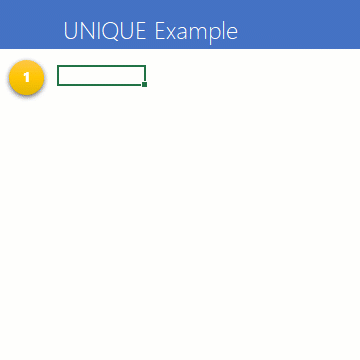What is the syntax of UNIQUE function?

Simplified syntax:

=UNIQUE(list)

With options:

=UNIQUE(list, data is across the columns?, do you want values occurring just once?)

Give me few examples of UNIQUE function?

Sure. Here are few more practical examples of UNIQUE function.

• UNIQUE(data[department])
List out all department, just one row per department.
• UNIQUE(FILTER(data[department], data[age]>30))
List out all departments where staff aged >30 work.
• UNIQUE({1;1;2;3;4;4;5;6;7;7;7;8;9;0;0}, FALSE, TRUE)
Return the numbers that occurred just once – ie  {2;3;5;6;8;9}

## SORT() Fact Sheet

What does SORT() do?

SORT() function sorts a list or data by the column number specified in ascending or descending order.

Show me a demo of SORT?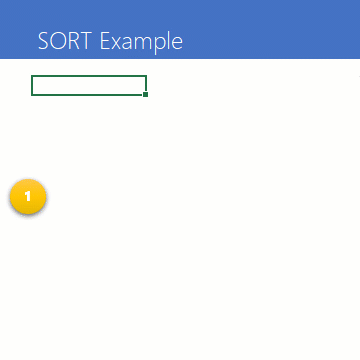What is the syntax of SORT function?

Common usage:

=SORT(list)

With options:

=SORT(list, column number, ascending or descending order, do you want to sort across the columns instead?)

Give me few examples of SORT function?

Here are a few more examples of SORT function:

• SORT(data[name])
Sorts all the names in data in ascending order (the default order)
• SORT(data, 6, -1)
Sorts all data by salary (column 6)  in descending order (-1)
• SORT(FILTER(data, data[manager]=”Carla”),4)
Shows all staff data that report to Carla in ascending order of age (column 4).

How does SORT break ties?

Let’s say you are sorting the staff data by age with the formula SORT(data, 4). In this case, if two employees have same age, then SORT will present them in the order as per original data. So if Bill and Jill both are 19, but they are listed Bill first in data, that is how SORT will show the result too.

How to use another column to break ties when SORTing?

In such cases, you can use the next function – SORTBY. This accepts multiple criteria to break ties.

## SORTBY() Fact Sheet

What does SORTBY() do?

SORTBY() sorts a list (or table) by a set of criteria lists. You can use this to break ties or defined multi-level sorting criteria (for ex: sort by department and age).

Show me a demo of SORTBY?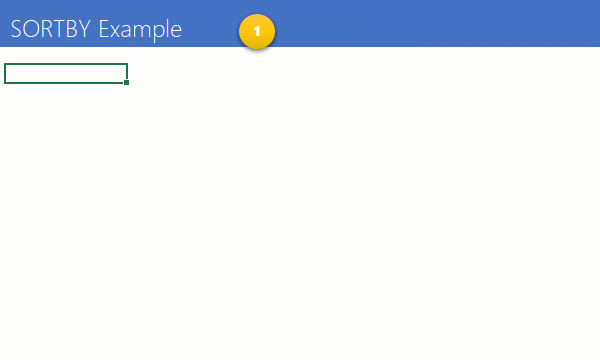What is the syntax of SORTBY function?

SORTBY(list, criteria list 1, sort order 1, criteria list 2, sort order 2…)

Give me few examples of SORTBY  function?

SORTBY opens up lots of possibilities to analyze and present data in meaningful manner. Here are few real-world scenarios.

• SORTBY(data, data[Department],1, data[Salary],-1)
Sort data by department in A-Z order and then Salary in descending order.
• SORTBY(data[name], data[Department],1, data[Salary],-1)
Sort data by department in A-Z order and then Salary in descending order, but just show the names.
• SORTBY(data, data[Department]=”HR”, -1, data[Name],1)
Shows all employees in HR department on top and rest underneath in alphabetical order.

## SEQUENCE() Fact Sheet

What does SEQUENCE() do?

SEQUENCE() function generates a sequence of numbers in rows or columns or both. You can use this to make running numbers. While this may not seem all that helpful, SEQUENCE opens up doors for creating elegant and powerful solutions for your data analysis needs.

Show me a demo of SEQUENCE?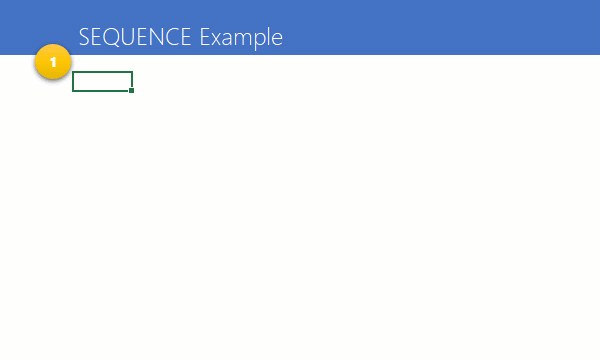What is the syntax of SEQUENCE function?

Common usage:

=SEQUENCE(count)

With options:

=SEQUENCE(row count, column count, starting number, step by)

Give me few examples of SEQUENCE function?

Here are a few more examples of SEQUENCE function:

• SEQUENCE(10)
Generates numbers 1 to 10 and spills them in to 10 cells.
• SEQUENCE(100)<=10
Generates 100 values, with first 10 as TRUE and others as FALSE
• FILTER(SORT(data, 6, -1), SEQUENCE(100)<=10)
Shows data for top 10 employees by salary.
• SORTBY(data, SEQUENCE(100), -1)
Prints data in reverse order (by sorting the sequence of 100 numbers in descending order)

## RANDARRAY() Fact Sheet

What does RANDARRAY() do?

RANDARRAY() makes a list of random numbers. Just as SEQUENCE(), RANDARRAY() too doesn’t seem like a useful function, until you need it.

Show me a demo of RANDARRAY?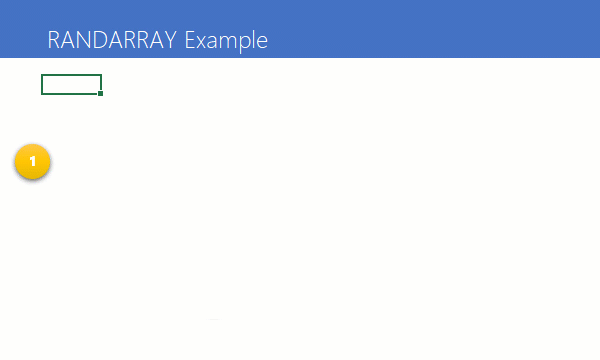What is the syntax of RANDARRAY function?

Common usage:

RANDARRAY(count)

With options:

RANDARRAY(row count, column count, starting number, ending number, do you want just random integers?)

Give me few examples of RANDARRAY function?

Here are a few real-world examples of RANDARRAY.

• RANDARRAY(10,,1,100,TRUE)
Generate 10 random integers between 1 to 100
• UNIQUE(RANDARRAY(10,,1,100,TRUE))
Generates 10 random integers between 1 to 100 and removes any duplicates in them.
• SORTBY(data, RANDARRAY(100))
Shuffles the data in random order

## How Dynamic Arrays interact with other Excel features?

DA functions and spill ranges play well with most Excel functionalities. I have made a few notes and comments about individual features below.

### Spill Ranges in other formulas

You can refer to spill ranges (output generated by Dynamic Array functions) using the # operator.

For example, let’s say you have a formula in cell B7 to filter all salaries of people reporting to Carla.

You can refer to the entire spilled range in other situations using # operator like this.

• SUM(B7#) – sums up all salaries listed in the cell B7 and down. This formula will automatically adjust if either data or filter conditions change.
• AVERAGE(B7#) – similar to SUM, but calculates average salary
• COUNTIFS(B7#, “>100000”) – counts number of values in the spill range (B7#) greater than \$100,000

See this illustration to understand how to work with spill ranges in other formulas.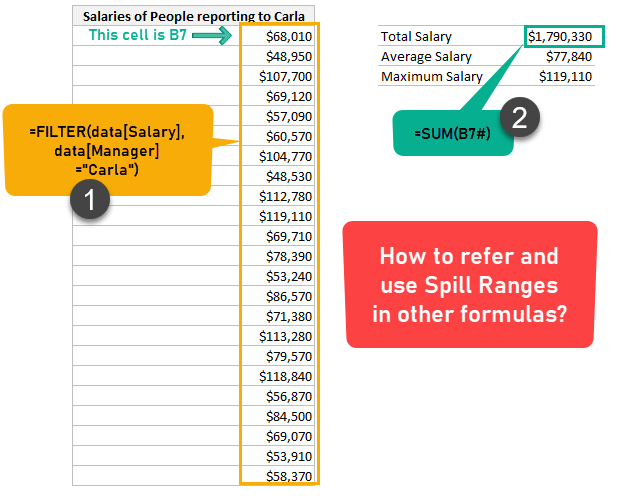### Named ranges and DA Functions

You can create named ranges that refer to Spill range. You can also use named ranges inside DA functions. Everything works just as smoothly.

For example,

1. We can create a named range called HighPerformers that refers to the formula =FILTER(data, data[Rating]>=5). See this illustration.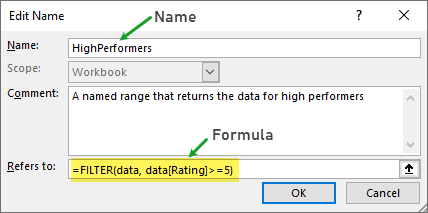2. We can then use this named range in other formulas (or situations). To count number of high performers, we can use =ROWS(HighPerformers)When you combine the DA functions with array processing power of INDEX, you can solve some gnarly business problems easily.

### Conditional Formatting Dynamic Arrays

As of April 2020, Conditional formatting doesn’t really recognize dynamic spill ranges. This means, when you create a CF rule to be applied to an entire spill range, even though Excel let’s you enter B7#, it will automatically convert the range to physical address (for eg. \$B\$7:\$B\$19).

So if your data or formula changes, the CF rules won’t automatically extend.

### Dynamic Arrays in Data Validation

You can use # operator when referring to dynamic array range with data validation rules.

For example, you can set a data validation drop down list in a cell to show all department names in ascending order using below method:

1. In an empty cell (say AH6), write the formula =SORT(UNIQUE(data[Department]))
2. Now, select the cell where you want to apply data validation, go to Data > Validation and select the type as List.
3. Set list source as \$AH\$6#
4. You can select departments from your validation list.

See this illustration on how this can be useful.### Charting & Dynamic Arrays

As of April 2020, Excel charts do not recognize spill range operators. This means, when you create a chart from spilled range, it will not automatically extend if the data / formula changes.

Here is a quick demo of this broken chart behavior.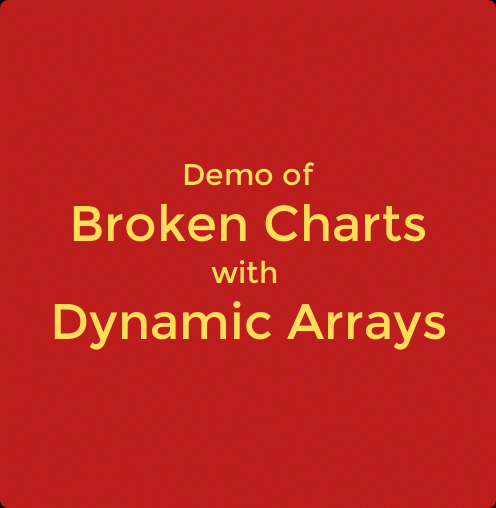## Dynamic Array Functions – Full Introduction Video

Coming soon…

I will be uploading a detailed video with full introduction to DA functions and examples soon. Bookmark this page and come back later this week.

## Example Workbook

I made a full example workbook with sample data, several formulas, interaction details and more. Please use below button to download the file.

Note: It will work ONLY if you have Dynamic Array feature in Excel 365.

## Additional Resources on DA Functions

• Dynamic Arrays in the traditional Excel world [Microsoft]
• Overview of Dynamic Array functions [Excel Campus]
• Excel Dynamic Arrays – Straight to the point – Book by Bill Jelen [MrExcel]
• Joe McDaid, Program Manager at Microsoft Explains the DA functionality – Video

## Dynamic Array Functions – Quick Course NEW

I am designing a 3 hour video course with more than 50 examples of Dynamic Array functions. This will be an beginner to advanced level course for people wanting to get more out of the DA features. This course is nearly ready and will be launching in 3rd week of April.

If you want to know more about it, please join the waiting list using below form. I will email you once we are ready.The post Excel Dynamic Array Functions – What are they, how to use them, Examples and FAQs appeared first on Chandoo.org – Learn Excel, Power BI & Charting Online.